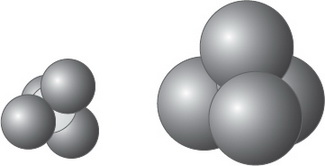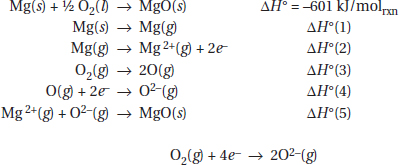# AP Chemistry Practice Test 35

### Test Information12 questions18 minutes

1. Fluorine has a normal boiling point of 85 K, and chlorine boils at 239 K. The Cl2 molecule is much larger than F2 (atomic radius is 99 pm for chlorine and is 64 pm for fluorine). Which is the best reason for the large difference in boiling points?

2.Space-filling representations of carbon tetrachloride and carbon tetrabromide are shown above. The carbon can be seen in the carbon tetrachloride and is hidden by the bromine in carbon tetrabromide. Which of the following is the most reasonable statement? (Assume that the temperatures of CCl4 and CBr4 are the same in these comparisons.)

`Questions 3-7 refer to the following information.Questions refer to the following formation equation for the reaction synthesizing RbBr(s). This reaction can be separated into a series of steps.`

3. Using Hess's law, which of the following combinations will give the enthalpy for the above reaction?

4. When 1.00 mol of O2(g) is formed from MgO(s) in the reaction above, how much heat is released or absorbed?

5. Which of the steps in the table above are endothermic?

6. Noting that this reaction goes to completion, producing MgO from the elements, and using the overall chemical equation to estimate the entropy change for this process, which of the following statements is correct?

7. If 1.0 g of magnesium and 1.0 g oxygen are reacted, what will be left in measurable amounts (more than 0.10 mg) in the reaction vessel?

`Questions 8-11 refer to the following information.Questions refer to the galvanic cells represented by cell diagrams and their corresponding cell voltages listed below.Galvanic CellCell DiagramCell Voltage1Ti | Ti2+(1.0 M) || Co2+(1.0 M) | Co1.352V | V2+(1.0 M) || Co2+(1.0 M) | Co0.903Ti | Ti2+(1.0 M) || V2+(1.0 M) | V?The chemical reaction occurring in galvanic cell 2 isV + Co2+ → V2+ + Co`

8. What is the standard cell potential for galvanic cell × depicted in the table above?

9. What is the chemical reaction under study in galvanic cell 3?

10. If the concentration of Ti2+ is changed from 1.0 M to 0.1 M in the galvanic cells above, what will happen to the observed cell voltages in galvanic cells 2 and 3?

11. Rank the three metals from the metal that is easiest to oxidize to the metal that is the most difficult to oxidize.

12. The first ionization energy of sodium is 496 kJ/mol, and its atomic radius is 186 pm.

Based on the information above and your knowledge of periodic trends, which values are the most reasonable for the radius and first ionization energy of the magnesium atom?